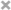## 特点概括

• 递归了划分数据：分而治之。Recursively partition the data: Divide and Conquer。
• 查询时间为：$$O(log n)$$(with constants exponential in dimension)
• 随着数据维数的增加，基于树的ANN其表现性能会急剧的下降，Performance degrades with high-dimensional data。
• 需要的存储开销很大，Large storage needs，因为需要存储树结构(?)。
• 在运行的时候，需要保存原始数据，Original data is required at run-time。同样会增加内存的开销。

• 数据库中的每一个item都被用一个编码来表达。Each item in database represented as a code。
• 可以极大的降低内存空间。Significant reduction in storage。
• 查询时间为：$$O(1)$$或是线性的。Expected query time: O(1) or sublinear in n。
• Compact codes preferred。

## 准召权衡

• 如果想要得到较高的精度，则需要较长的编码。For high precision, longer codes (i.e. large $$m$$) preferred。
• 编码长度m增长的话，则item碰撞的概率会成倍的减小，从而导致召回率下降。 Large m reduces the probability of collision exponentially → low recall
• 为了得到较高的召回率，则需要多个哈希表。Many tables (large L) necessary to get good recall → Large storage